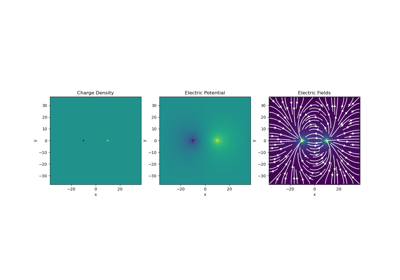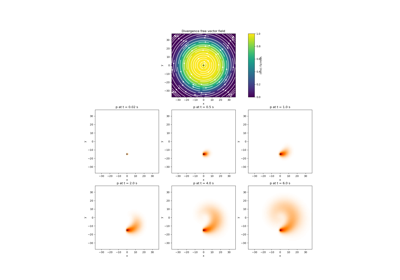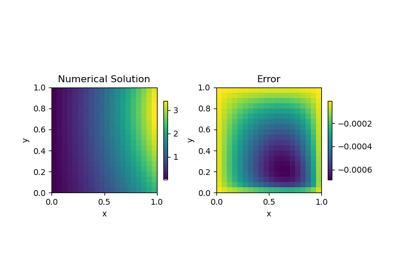# Solving PDEs#

Here we show how the discretize package can be used to solve partial differential equations (PDE) numerically by employing the finite volume method. To solve a PDE numerically we must complete the following steps:

1. Formulate the problem; e.g. the PDE and its boundary conditions

2. Apply the weak formulation by taking the inner product of each PDE with a test function

3. Formulate a discrete set of equations for the inner products according to the finite volume method

4. Use the discrete set of equations to solve for the unknown variable numericallyGauss’ Law of Electrostatics

Gauss' Law of Electrostatics